# Area of a square inscribed in a circle which is inscribed in a hexagon in C Program?

Here we will see the area of a square which in inscribed in one circle and that circle is inscribed in a hexagon. The side of the square is ‘a’. The radius of the circle is ‘r’, and the side of the hexagon is ‘A’. The diagram will be like below.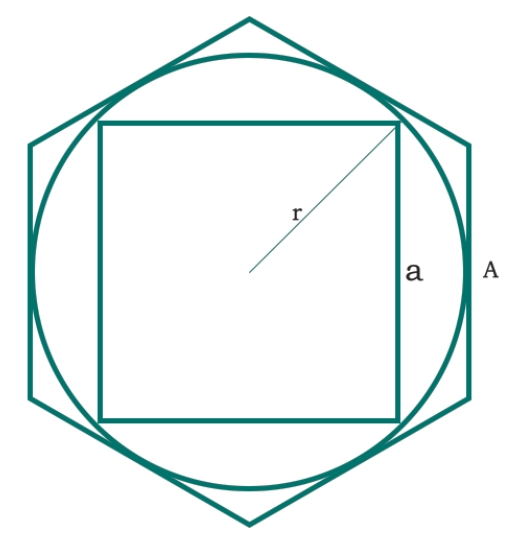We know that the radius of a circle inscribed in a hexagon is −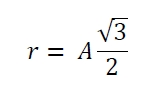Also the radius of the circle is half of the diagonal of the square. So −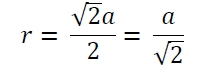Then we can say −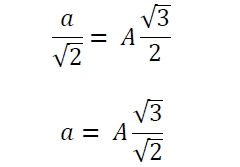Then the area will be −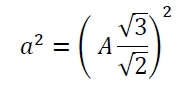## Example

#include <iostream>
#include <cmath>
using namespace std;
float area(float A) { //A is the side of the hexagon
if (A < 0) //if the value is negative it is invalid
return -1;
float area = (A*A) * float(3.0/2.0);
return area;
}
int main() {
float side = 5;
cout << "Area is: " << area(side);
}

## Output

Area is: 37.5

Updated on: 20-Aug-2019

47 Views